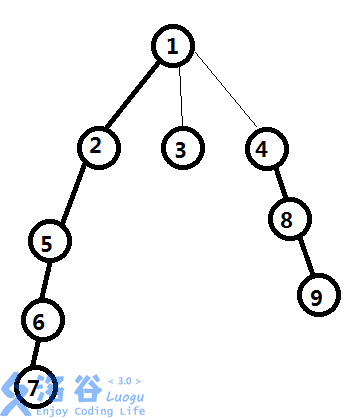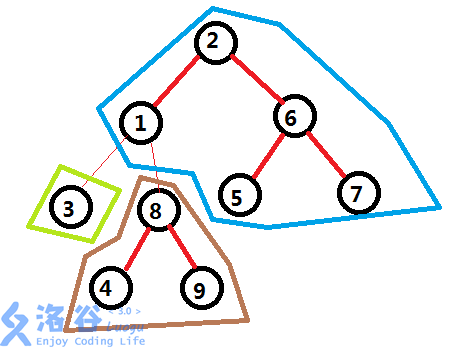# 柒葉灬 的博客# 柒葉灬 的博客

### LCT总结（个人笔记）

posted on 2019-03-10 14:46:24 | under 专题总结 |

# LCT总结

(或许还有长链剖分

## 实链剖分

1. 怎么保证跳的实链的次数不会过大？
2. 怎么跳到每条链的顶端的父亲？

### 第二个问题：怎么跳到每条链的顶端的父亲？

1. 左儿子深度都比当前点
2. 右儿子深度都比当前点

splay当中最左边的数就是链的顶端了。mmp为什么写了半天的概念

$up,down$ 以及 $pushr$ (翻转)与普通 $splay$ 一样。

bool noroot(int x){
return son[fa[x]]==x||son[fa[x]]==x;
}//如果不是splay的根返回true;

### rotate操作

void rotate(int x){
int y=fa[x];
int z=fa[y];
int k=(son[y]==x);
if(noroot(y))son[z][(son[z]==y)]=x;
fa[x]=z;
son[y][k]=son[x][k^1];
fa[son[x][k^1]]=y;
son[x][k^1]=y;
fa[y]=x;
up(y);up(x);
}

### splay操作

void splay(int x){
int u=x,top=0;
stk[++top]=u;
while(noroot(u))stk[++top]=u=fa[u];
while(top)down(stk[top--]);
//即先down完之后再splay
while(noroot(x)){
int y=fa[x];
int z=fa[y];
if(noroot(y))
(son[z]==y)^(son[y]==x)?rotate(x):rotate(y);
rotate(x);
}
}

### access操作

void access(int x){
for(int y=0;x;y=x,x=fa[x])
splay(x),son[x]=y,up(x);
}

1. $splay(x)$ ：把x旋转到根
2. $son[x]=y$ ：之前的实边被覆盖，实儿子变成y。

### makeroot操作

void makeroot(int x){
access(x);splay(x);pushr(x);
}

### findroot操作

int findroot(int x){
access(x);splay(x);
while(son[x])down(x),x=son[x];
splay(x);//保证复杂度
return x;
}

### split操作

void split(int x,int y){
makeroot(x);access(y);splay(y);
//直接对y节点进行询问即可
}

bool link(int x,int y){
makeroot(x);
if(findroot(y)==x)return false;
fa[x]=y;//直接连接虚边
return true;
}

void link(int x,int y){
makeroot(x);
fa[x]=y;
}

### cut操作

bool cut(int x,int y){
makeroot(x);
if(findroot(y)!=x||fa[y]!=x||son[y])return false;
son[x]=fa[y]=0;
up(x);
return true;
}

void cut(int x,int y){
split(x,y);
son[y]=fa[x]=0;
up(y);
}

LCT专题L

### LCT维护子树信息

$LCT$ 实际上也可以维护子树的信息

LCT专题K

END